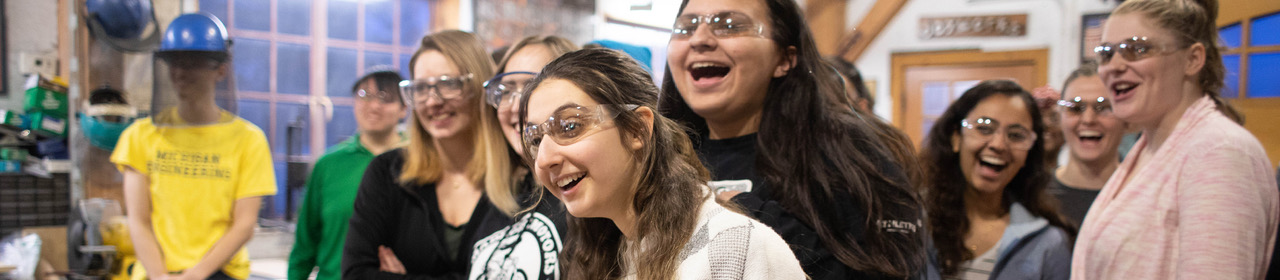•## MSE335 : Kinetics and Transport in Materials Engineering

##### Application of basic principles of molecular transport and mass, energy and momentum balance to the solution of heat, diffusion and fluid flow problems relevant to materials processing. Introduction to radiative heat transfer. Empirical approaches to and dimensional analysis of complex transport problems including convection, turbulence and non-Newtonian flow.

Prerequisites: Math 216 and MSE 220 or 250

### Course Topics:

1. Systems of units.
2. Balance laws: mass, species, momentum, thermal energy, mechanical energy.
3. Application of balance laws to flow in pipes and tanks.
4. Differential forms of balance laws.
5. Constitutive laws for molecular transport: Fick's law, Fourier's law, Newton's law.
6. Boundary conditions for transport problems.
7. Combining balance, molecular transport and convection.
8. Solving one-dimensional transport problems using differential equations.
9. Solving higher dimensional transport problems using numerical methods.
10. Deriving engineering (net) transport equations from microscopic equations.
11. Radiative Heat Transfer: Stephan-Boltzmann Law, black bodies, gray bodies, view factors.
12. Turbulent flow.
13. Dimensional analysis and dimensionless parameters.
14. Phenomenological transfer coefficients (heat, momentum, mass).
15. Nonlinear constitutive laws for transport; phenomenological non-Newtonian flow laws.
16. Kinetic theories of transport: Chapman-Enskog Theory, Eyring Free Volume Theory, Debye Theory, theory of electronic thermal conduction.

### Course Objectives:

1. To understand and be able to apply the basic equations of molecular transport (Fourier, Fick and NewtonÕs Laws).
2. To utilize the equations of mass, energy and momentum balance to analyze transport problems.
3. To combine (1) and (2) above in solving problems in the presence and absence of convection.
4. To become familiar with the issues associated with turbulent flow and be able to utilize phenomenological approaches for analyzing turbulent flows.
5. To learn representative kinetic theories for calculating transport coefficients in solids, liquids and gases.
6. To understand the importance of dimensional analysis and the role of dimensionless quantities in determining transitions in physical behavior and quantifying empirically determined boundary conditions.

### Course Outcomes:

1. Students will be able to convert a transport problem into a set of differential equations for further analysis.
2. Students will be able to calculate the power requirements for a piping network given data for friction factors and loss coefficients.
3. Students will be able to use ordinary differential equations to determine how temperature and chemical composition vary with time in a simple reactor.
4. Students will be able to apply linear constitutive laws to calculate effective transfer coefficients through composite structures.
5. Students will be able to compute heat transfer between radiating bodies.
6. Students will be able to use kinetic theories of transport to compute the temperature dependence of transport coefficients.
7. Students will be able to express spatially dependent transport problems in terms of partial differential equations and solve these equations analytically in cases that can be reduced to one spatial dimension.
8. Students will be able to analyze one-dimensional solidification using partial differential equations in order to predict cooling rates and solidification times.
9. Students will be able to apply dimensional analysis to physical problems.
10. Students will be able to calculate dimensionless parameters associated with transport and use these to compute transfer coefficients.
11. Students will be able to describe non-Newtonian behavior using phenomenological constitutive laws.

### Assessment Tools:

1. Four (4) tests, each on one aspect of transport.
2. Weekly problem sets (11 in all).